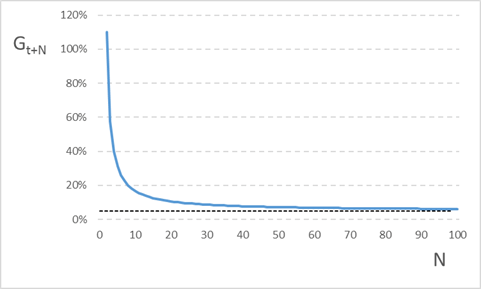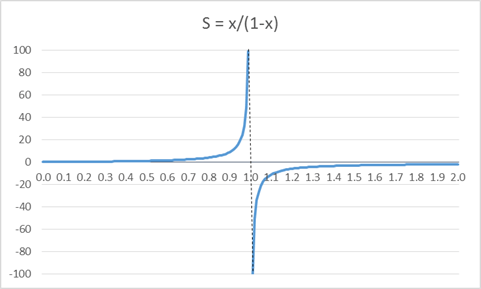Economists are familiar with constant discounted growth:and with the fact that, for Pt to be positive, R must be bigger than G. Indeed R-G is all the rage these days.

But why on earth in that the case? How can the sum of a stream of dividends (or incomes) growing at G and discounted at R be negative if G>R? If anything, as dividends grow faster than they get discounted, the elements of the sum get larger and larger. How can the sum of larger and larger positive numbers be negative?

As an example, take the following stream: D=(1.1, 2.2, 3.5, 4.9, 6.4, 8.0, 9.8, 11.8, 14.0, 16.3, …). The stream is such that the Nth element is equal to N(1+R)N, with R=5%. Therefore, the Nth element of the stream of successive growth rates is N(1+R)/(N-1)-1=(1+RN)/(N-1). Though not constant, growth rates are always larger than R, and converge to R as N grows:Since Gt+N>R for all N, we must conclude that the value of a company that distributes D over time is negative. The value is equal to the sum of discounted dividends, which, by construction, is the series of natural numbers: DD=(1, 2, 3, 4, 5, 6, 7, 8, 9, 10, …). So here is the mindboggling conclusion: the sum of natural numbers is negative.

What?

Of course, we are talking about the infinite sum of natural numbers. Because the sum of the first N natural numbers is: S = 1+2+3+…+(N-2)+(N-1)+N = N+(N-1)+(N-2)+…+3+2+1 and, summing the elements pairwise, we have 2S = (N+1)+(N+1)+…+(N+1) = N(N+1), and therefore S = N(N+1)/2. So S is, as it should be, a natural number, which gets bigger and bigger as N grows. How can it possibly become a negative number as N goes to infinity? It can’t be true. But here it is. Let’s define three infinite series:

S = 1 + 2 + 3 + 4 + 5 + 6 + …

S1 = 1 – 1 + 1 – 1 + 1 – 1 + …

S2 = 1 – 2 + 3 – 4 + 5 – 6 + …

S1 is known as Grandi’s series and its sum is either 0 or 1, depending on whether we take an even or odd number of elements. So let’s take the average: S1 = 1/2.

To calculate S2, let’s sum it to itself. We have 2S2 = 1 + (–2+3–4+5–6+…) + (1–2+3–4+5–6+…) and, summing pairwise the elements in the two brackets, we have 2S2 = S1. Hence S2 = 1/4.

Then we have S – S2 = (1+2+3+4+5+6+ …) – (1–2+3–4+5–6+…) = 4+8+12+… = 4S, again summing pairwise the elements in the two brackets. Hence, S–1/4 = 4S, and therefore S = -1/12.

There we go: the infinite sum of natural numbers is equal to -1/12. That is the sum of the elements of DD, the series of discounted dividends. Therefore a share of a company that distributes dividend stream D over time must cost nothing: if you buy it, you actually get it for free, plus you get 8.33 cents.

If you think I am joking, take a look at this:

If, like me, you do not believe that S1=1/2 – I think it is zero – the proof breaks down. But a more rigorous proof confirms the result.

Of course, I am joking about the valuation. In economics, we exclude G>R because it leads to nonsensical results. And we know that, for finite N, G can be bigger than R. And that’s it.

But the mathematical result remains. Like constant discount growth, it is a special case of S = x+x2+x3+… = x/(1-x), which is positive for 0<x<1, negative for x>1 and undefined for x=1. This is what S looks like in the neighbourhood of 1:As in constant discounted growth, if 0<x<1 (corresponding to R>G) the series is the sum of smaller and smaller positive numbers, i.e. it is convergent and well behaved. For example, it solves the most famous of Zeno’s paradoxes: Achilles does reach the tortoise in finite time. But if x>1 the series is divergent and ill behaved: it is the sum of larger and larger positive numbers, but is, apparently and puzzlingly, a negative number, tending to -1. And for x=1 – for which S is an infinite sum of 1s – the series is undefined, tending to plus infinity from the left, but to minus infinity from the right.

If we differentiate both sides of S with respect to x, we get dS = 1+2x+3x2+… = 1/(1-x)2, which, unlike S, is always positive and, like S, is undefined for x=1:Like S, dS is convergent and well behaved for 0<x<1, but divergent and ill behaved for x>1, as it is the sum of larger and larger positive numbers and at the same time, apparently and puzzlingly, a small positive number, tending to zero (although, mercifully, it is not a negative number). But for x=1 the left hand side of dS is none other than our infinite sum of natural numbers, which is therefore – just like the infinite sum of 1s – undefined, although at least it tends to plus infinity from both sides. In fact, the infinite sum of natural numbers is simply an infinite sum of infinite sums of 1s.

So how can it possibly be equal to -1/12? And what does that mean? To me it means that infinity is only a convenient but senseless concept.

At the end of the video, Brady asks Tony: “So if I do 1+2+3+4 up to a googolplex…”. Tony interjects and says smilingly: “You get a big number. You won’t get anything like -1/12. You have to go to infinity, Brady”.

You can’t, Tony. There is no infinity.

This site uses Akismet to reduce spam. Learn how your comment data is processed.